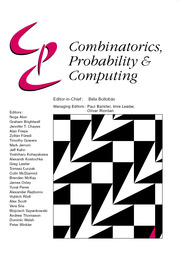HomeCombinatorics, Probability and Computing

# On the Difference of Expected Lengths of Minimum Spanning Trees

Published online by Cambridge University Press:  01 May 2009

Corresponding

## Abstract

An exact formula for the expected length of the minimum spanning tree of a connected graph, with independent and identical edge distribution, is given, which generalizes Steele's formula in the uniform case. For a complete graph, the difference of expected lengths between exponential distribution, with rate one, and uniform distribution on the interval (0, 1) is shown to be positive and of rate ζ(3)/n. For wheel graphs, precise values of expected lengths are given via calculations of the associated Tutte polynomials.

Type
Paper
Information
Combinatorics, Probability and Computing , May 2009 , pp. 423 - 434

## Access options

Get access to the full version of this content by using one of the access options below.

## References

Avram, F. and Bertsimas, D. (1992) The minimum spanning tree constant in geometric probability and under the independent model: A unified approach. Ann. Appl. Probab. 2 113130.CrossRefGoogle Scholar
Benedict, C. P. (1969) On self-dualisms in graphs and networks. PhD dissertation, University of Waterloo, Waterloo, Ontario, Canada.Google Scholar
Benjamin, A. T. and Yerger, C. R. (2006) Combinatorial interpretations of spanning tree identities. Bull. Inst. Combin. Appl. 47 3742.Google Scholar
Beveridge, A., Frieze, A. and McDiarmid, C. (1998) Minimum length spanning trees in regular graphs. Combinatorica 18 311333.CrossRefGoogle Scholar
Bollobás, B. (2001) Random Graphs, 2nd edn, Cambridge University Press.CrossRefGoogle Scholar
Bollobás, B. (2002) Modern Graph Theory, Springer.Google Scholar
Cayley, A. (1889) A theorem on trees. Quart. J. Pure Appl. Math. 23 376378.Google Scholar
Fill, J. A. and Steele, J. M. (2005) Exact expectations of minimal spanning trees for graphs with random edge weights. Stein's method and applications, Lect. Notes Ser. Inst. Math. Sci. Natl. Univ. Singap., Singapore University Press, Singapore, 5169180.Google Scholar
Flaxman, A. D. (2007) The lower tail of the random minimum spanning tree. Electron. J. Combin. 14 #3.Google Scholar
Frieze, A. M. (1985) On the value of a random minimum spanning tree problem. Discrete Appl. Math. 10 4756.CrossRefGoogle Scholar
Frieze, A. M. and McDiarmid, C. J. H. (1989) On random minimum length spanning trees. Combinatorica 9 363374.CrossRefGoogle Scholar
Frieze, A. M., Ruszinkó, M. and Thoma, L. (2000) A note on random minimum length spanning trees. Electron. J. Combin. 7 #5.Google Scholar
Gamarnik, D. (2005) The expected value of random minimal length spanning tree of a complete graph. In Proc. 16th Annual ACM–SIAM Symposium on Discrete Algorithms, pp. 700–704.Google Scholar
Hutson, K. and Lewis, T. M. (2007) The expected length of a minimum spanning tree of a cylinder graph. Combin. Probab. Comput. 16 6383.CrossRefGoogle Scholar
Janson, S. (1993) Multicyclic components in a random graph process. Random Struct. Alg. 4 7184.CrossRefGoogle Scholar
Janson, S. (1995) The minimal spanning tree in a complete graph and a functional limit theorem for trees in a random graph. Random Struct. Alg. 7 337355.CrossRefGoogle Scholar
McDiarmid, C. (1989) On the method of bounded differences. In Surveys in Combinatorics, Vol. 141 of London Mathematical Society Lecture Note Series, Cambridge University Press, pp. 148188.Google Scholar
Myers, B. R. (1971) Number of spanning trees in a wheel. IEEE Trans. Circuit Theory CT-18 280282.CrossRefGoogle Scholar
Penrose, M. (1998) Random minimum spanning tree and percolation on the n-cube. Random Struct. Alg. 12 6382.3.0.CO;2-R>CrossRefGoogle Scholar
Sedlacek, J. (1970) On the skeletons of a graph or digraph. In Proc. Calgary International Conference on Combinatorial Structures and their Applications, Gordon and Breach, pp. 387–391.Google Scholar
Smith, C. A. B. and Tutte, W. T. (1950) A class of self-dual maps. Canad. J. Math. 2 179196.CrossRefGoogle Scholar
Steele, J. M. (1987) On Frieze's ζ(3) limit for lengths of minimal spanning trees. Discrete Appl. Math. 18 99103.CrossRefGoogle Scholar
Steele, J. M. (2002) Minimum spanning trees for graphs with random edge lengths. In Mathematics and Computer Science II: Algorithms, Trees, Combinatorics and Probabilities, Birkhäuser, pp. 223245.CrossRefGoogle Scholar
Tutte, W. T. (1950) Squaring the square. Canad. J. Math. 2 197209.CrossRefGoogle Scholar
Welsh, D. (1999) The Tutte polynomial. Random Struct. Alg. 15 210228.3.0.CO;2-R>CrossRefGoogle Scholar

### Full text views

Full text views reflects PDF downloads, PDFs sent to Google Drive, Dropbox and Kindle and HTML full text views.

Total number of HTML views: 0
Total number of PDF views: 36 *
View data table for this chart

* Views captured on Cambridge Core between September 2016 - 26th January 2021. This data will be updated every 24 hours.

Hostname: page-component-898fc554b-q2rfn Total loading time: 0.26 Render date: 2021-01-26T10:04:21.887Z Query parameters: { "hasAccess": "0", "openAccess": "0", "isLogged": "0", "lang": "en" } Feature Flags: { "shouldUseShareProductTool": true, "shouldUseHypothesis": true, "isUnsiloEnabled": true, "metricsAbstractViews": false, "figures": false, "newCiteModal": false }

# Send article to Kindle

Note you can select to send to either the @free.kindle.com or @kindle.com variations. ‘@free.kindle.com’ emails are free but can only be sent to your device when it is connected to wi-fi. ‘@kindle.com’ emails can be delivered even when you are not connected to wi-fi, but note that service fees apply.

Find out more about the Kindle Personal Document Service.

On the Difference of Expected Lengths of Minimum Spanning Trees
Available formats
×

# Send article to Dropbox

To send this article to your Dropbox account, please select one or more formats and confirm that you agree to abide by our usage policies. If this is the first time you use this feature, you will be asked to authorise Cambridge Core to connect with your <service> account. Find out more about sending content to Dropbox.

On the Difference of Expected Lengths of Minimum Spanning Trees
Available formats
×

# Send article to Google Drive

To send this article to your Google Drive account, please select one or more formats and confirm that you agree to abide by our usage policies. If this is the first time you use this feature, you will be asked to authorise Cambridge Core to connect with your <service> account. Find out more about sending content to Google Drive.

On the Difference of Expected Lengths of Minimum Spanning Trees
Available formats
×
×